Analysis of underwater decoupling properties of a locally resonant acoustic metamaterial coating
Huang Ling-Zhi, Xiao Yong, Wen Ji-Hong†,, Yang Hai-Bin, Wen Xi-Sen
Vibration and Acoustics Research Group, Laboratory of Science and Technology on Integrated Logistics Support, College of Mechatronic Engineering and Automation, National University of Defense Technology, Changsha 410073, China

† Corresponding author. E-mail: wenjihong@vip.sina.com

Project supported by the National Natural Science Foundation of China (Grant Nos. 51305448 and 51275519).

Abstract
Abstract

This paper presents a semi-analytical solution for the vibration and sound radiation of a semi-infinite plate covered by a decoupling layer consisting of locally resonant acoustic metamaterial. Formulations are derived based on a combination use of effective medium theory and the theory of elasticity for the decoupling material. Theoretical results show good agreements between the method developed in this paper and the conventional finite element method (FEM), but the method of this paper is more efficient than FEM. Numerical results also show that system with acoustic metamaterial decoupling layer exhibits significant noise reduction performance at the local resonance frequency of the acoustic metamaterial, and such performance can be ascribed to the vibration suppression of the base plate. It is demonstrated that the effective density of acoustic metamaterial decoupling layer has a great influence on the mechanical impedance of the system. Furthermore, the resonance frequency of locally resonant structure can be effectively predicted by a simple model, and it can be significantly affected by the material properties of the locally resonant structure.

1. Introduction

Attaching an elastic decoupling layer to a vibrating structure immersed in water represents a common way to reduce the sound radiation of the vibrating structure. This treatment is effective to isolate the fluid from vibrations of the structure by reason of mismatching between water and decoupling layer and mismatching between decoupling layer and the plate.

Sung has explored the use of air-voided elastomer decoupling layer in reducing noise of flexible plate. His research ensures that the amount of noise reduction increases as the thickness of the decoupling layer, the speed of dilatational wave, and dilatational wave’s loss factor increases, while the effects of shear wave speed are insignificant.

Berry and Foin have investigated the forced response and the sound radiation of decoupled plates with three-dimensional elasticity model. Their research shows that shear wave components in the decoupling material usually have little effect on the sound radiation in a heavy fluid. Wang’s experiment shows that calculation result of three-dimensional elasticity model and test results coincide well with each other.

In order to increase the effectivity of noise reduction, cavities with different shapes have always been embedded into decoupling layer with the increase of impedance mismatching. Tao et al. have investigated a sound radiation model of an infinite plate coved with a void decoupling layer. Their analysis indicates that primary mechanism of the noise reduction in their model is the vibration isolation in the decoupling layer. Zhu et al. make an experimental and numerical investigation about the sound radiation of the floating structures covered with chiral layer. Their results show that chiral layer can effectively reduce underwater sound radiated by the structure. Huang et al. have investigated decoupling mechanism of a viscoelastic decoupling layer with horizontal cylindrical cavities. Their study shows that the decoupling layer with horizontal cavities can greatly enhance the mechanical impedance and suppress the mean square velocity of vibrating structure. On the other hand, the decoupling layer with horizontal cavities has larger impedance mismatch with water than the decoupling layer without them, thus it brings more vibration transmission loss.

Although traditional decoupling layer has an excellent performance on noise attenuation at high frequencies, it should be mentioned that they have little effect at low frequencies. In some cases, noise radiated by structure with decoupling layer may increase at low frequencies.[4,6]

Locally resonant metamaterials (LRM) have been introduced by Liu et al. as a conceptual realization of an acoustic metamateria.[7,9] LRM has been used as an effective way to control subwavelength waves at low frequencies. In recent years, localized resonances in phononic crystals have been introduced to improve the low-frequency acoustic absorption of viscoelastic materials.[10,11] Zhao et al. investigated the acoustic absorption of viscoelastic slabs embedded with resonant sonic materials. Their theoretical and experimental studies indicate that locally resonant sonic materials have an outstanding performance of sound absorption. Wen et al. later used finite element method (FEM) to reveal the absorption mechanism. Their study presents that localized resonance leads to the absorption peak. Jiang et al. introduced woodpile structure into LRM, and used it in underwater acoustic absorbing material. Their structure shows an excellent performance on acoustic absorbing in their research. Duan et al. demonstrate that perfect absorption of elastic wave can be achieved by ultra-thin elastic meta-films when the boundary is a free space or a hard wall. And the pure imaginary effective mass density condition which is used for perfect absorption can be achieved by elastic metamaterials with large damping.

Much work has been conducted to investigate the sound absorption of the LRM, but little has been done about the noise reducing capability of the LRM when it is attached to the surface of vibrational underwater structure. In this paper, a semi-analytical formulation of the sound radiation from an underwater semi-infinite plate covered by a decoupling layer embedded with LRM is investigated. In the formulations, effective medium theory is adopted to obtain the equivalent properties of the decoupling layer with LRM, thereby elastic theory can be applied to the decoupling layer. In addition, the base plate in this work is dealt with classic plate theory. A solution of the vibration and sound radiation is derived from the composite system with simply supported boundary conditions. The proposed method is verified by the consistency between the predictions by FEM and the method in this paper. By using the mechanical impedance and modality theory, the vibration and sound radiation characteristics of the decoupling system are further examined.

2. Model and method
2.1. Effective material properties

Figure 1 shows the model of a viscoelastic polymeter layer embedded with singly periodic columniform locally resonant scatterer (LRS) under normal incidence of plane wave in the water. The radius of scatter’s core is r2, and the radius of scatter’s coating is r1. The cycle length of the periodic scatterers is d. The thickness of the slab is h2.

For brevity, the time-harmonic dependence of the form eiωt is assumed. The acoustic pressure of incident wave can be expressed as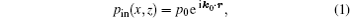where p0 is the amplitude of the incident wave, r is the position vector, and k0 is the wave vector in the fluid.

 Figure Option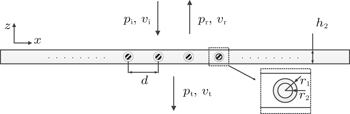Fig. 1. The model of plane wave transmission through an slab embedded with periodic LRS.

According to Bloch theorem for periodic systems, the discrete translational symmetry with period d in the x direction means the physical fields χ(x,z) satisfying the Bloch condition: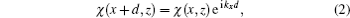where kx is the wave vector in the x direction.

In the incident acoustic field, the wave zone includes incident wave and reflected wave:where p1 is total sound pressure in incident acoustic field, pin is the sound pressure of the incident wave, and pr is the sound pressure of the reflected wave.

The sound pressure of the reflected wave can be expressed as: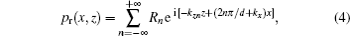where Rn is amplitude of the n-th expansion coefficient of the reflected wave.

The sound pressure of transmitted wave can be expressed as: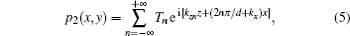where Tn is amplitude of the n-th expansion coefficient of the transmitted wave.

Using the FEM and Bloch theorem, the model of infinite structure with periodic cell can be constructed by just one cell with periodic boundary, and the equation of the acoustic-fluid-structure coupling can be expressed as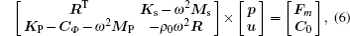where ρ0 is the density of the fluid, MP is the mass matrix of fluid, R is the matrix of fluid-solid interaction, Ms is the mass matrix of the solid, KP is the stiffness matrix of the fluid, Ks is the stiffness matrix of the solid, p is the vector of the sound pressure, u is the vector of the displacement, Fm is the vector of the node load, and C0, CΦ are the vectors of equivalent node load on the boundary.

It can be noted that the velocity v in systems satisfies the relation: v = iωu, so the amplitude of the sound pressure p1, the velocity v1 on the intersection plane of the viscoelastic polymeter slab with the incident acoustic field, and the amplitude of the sound pressure p2, the velocity v2 on the intersection plane of the viscoelastic polymeter slab with the transmission field and can be solved in Eq. (6). According to equivalent theory, the relation between p1, v1, and p2, v2 can be expressed with effective properties as: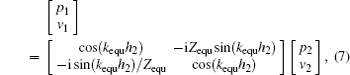where Zequ is the effective acoustic impedance of the slab, it can be expressed as: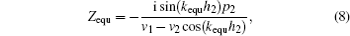kequ is the effective wave vector of the slab, it can be expressed as: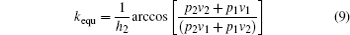and effective density ρequ is given by ρequ = Zequkequ/ω.

2.2. Vibration and sound radiation in the water

Figure 2 shows the model of sound radiation from a coated plate excited by a line force. The decoupling layer is a viscoelastic polymeter embedded with singly periodic columniform LRS as figure 1 shows. The thickness of base layer is h1, and the length of base layer in the x direction is Lx. The length of system in the direction perpendicular to xz plane is infinite. E1, ϑ1, and ρ1 are the Young modulus, the Poisson ratio, and the density of the base layer respectively. The boundaries of system on the lateral edges of the plate satisfy simply supported conditions. The line force is located on the base plate. The outer surface of the decoupling layer is inserted in an infinite rigid baffle with water on one side and air on the other side.

 Figure Option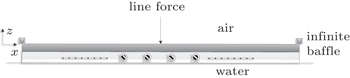Fig. 2. The model of sound radiation from a coated plate.

After decoupling layer is treated as effective homogeneous material, the dynamical equation of decoupled plate could be expressed with elastic theory as: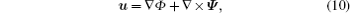where Φ represents longitudinal wave and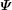represents transverse wave. According to some theories in literature,[2,18] transverse wave in homogenous decoupling layer has little influence on sound radiation of system, and decoupling layer is equivalent to a homogenous layer in this paper. Therefore, we omit transverse wave in the calculation. The equation of longitudinal wave in decoupling layer can be expressed as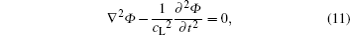where cL is equivalent longitudinal wave velocity. The equation of the base layer which is described with the classical plate theory can be expressed as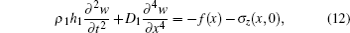where w is the displacement of the base plate in the z direction, D1 = E1h13/[12(1 − ϑ1)], f(x) is the line force, and σz(x,0) is the stress exerted by the decoupling layer on the plate in the z direction.

The boundary conditions for the base plate which is simply supported on the lateral sides are: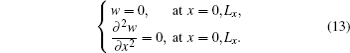Equation (13) allows the displacement of the base plate w to be expressed as trigonometric functions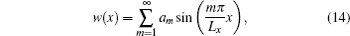where am is unknown coefficient of base plate’s displacement. The boundary conditions for decoupling layer on the lateral sides are: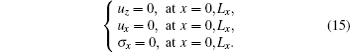The scalar potential of the decoupling layer can also be expressed in terms of similar trigonometric functions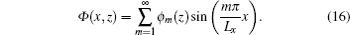Substituting Eq. (16) into Eq. (11) gives the following relation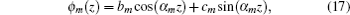where bm and cm are undetermined coefficients, and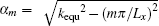. Therefore, the equation of displacement of the decoupling layer can be expressed as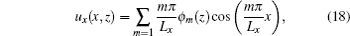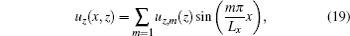where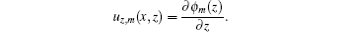Considering the relation of Lame constants in effective material: λ ≫ 2μ, the expression for the stress of decoupling layer in z direction could be written in the following form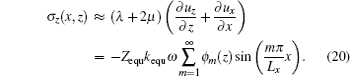The boundary conditions at the plate/decoupling layer interface can be expressed as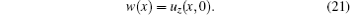The boundary conditions at the decoupling/water interface are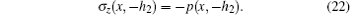This boundary conditions express the continuity of the stress between the plate and the decoupling layer. The acoustic pressure in the fluid half-space can be calculated by Rayleigh integral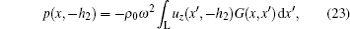where G is the acoustic Green function that satisfies a Neumann’s condition at z = −h2 in two-dimensional space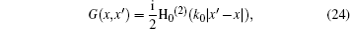where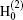is the zero-order Hankel function of the second kind, and ρ0 is the density of the fluid.

Substituting Eqs. (14), (19), and (20) into Eqs. (12), (21), and (22), multiplying the sin(mπx/Lx) on both sides of Eqs. (12) and (22), and integrating the equation in the range of [0,Lx], the following equations can be obtained: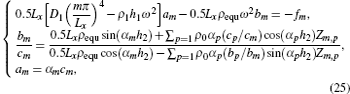where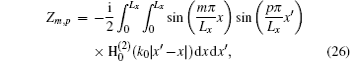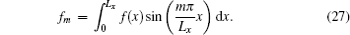Therefore, am, bm, and cm can be solved from Eq. (25), and the mean square velocity of base plate can be expressed asThe mean square velocity of the outer surface of decoupling layer is defined by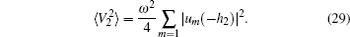The sound power radiated by outer surface of the system in the water is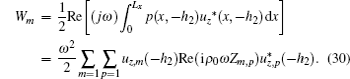3. Numerical results
3.1. The effect of noise reduction

In order to confirm the validity of the proposed method in this paper, the result calculated by FEM (calculated by Comsol4.4) is compared with the present method, as shown in Fig. 3. The base layer in Fig. 3 is made by steel (ρ1 = 7800 kg/m3, E1 = 210 Gpa, ϑ1 = 0.28) with dimensions Lx = 1.05 m, h1 = 30 mm. The decoupling layer has a thickness of h2 = 20 mm with main material’s longitudinal wave velocity cl_main = 1500 m/s, transverse wave velocity cs_main = 200 m/s, density density ρmain = 1050 kg/m3, and a loss factor ηmain = 0.15. The coating of the scatterers have a radius of r1 = 8.5 mm, with cl_coat = 1000 m/s, transverse wave velocity cs_coat = 6 m/s, density ρcoat = 1050 kg/m3, and a loss factor ηcoat = 0.05. The core of the scatterers have a radius of r2 = 5 mm, with Lame constants λcore = 42.3 Gpa, μcore = 14.9 Gpa, and ρcore = 11600 kg/m3. The amplitude of line force is 1 N/m.

Figure 3 shows sound power of the system calculated by paper’s method and Comsol4.4b. The results calculated by the two methods are matched perfectly below 900 Hz in Fig. 3(a) where the excited position is located at 0.5Lx.

In Fig. 3(b), there are some differences between two calculation results when the excited position is located at 0.1Lx and the excited frequencies are above 600 Hz. The reason for this phenomenon can be attributed to the boundary conditions of FEM which are simulated to simply supported conditions by fixing a point on the neutral plane of the model on each side, and the excited position which is too close to the boundary. With the excited frequency increasing and the wavelength being shorter, it is too hard to keep the boundary of the FEM model to the simply support conditions for the reason of the local stress in the coat layer which is near to the excited position. Although there are some differences between the calculation results when the excited position is near to the boundaries, it doesn’t affect investigating the decoupling mechanism of the layer with paper’s method.

 Figure Option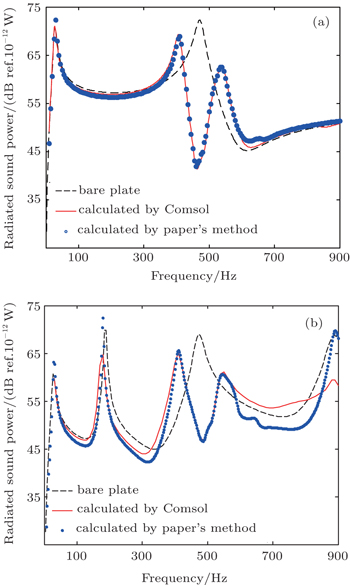Fig. 3. Sound power stimulated by line force at (a) 0.5Lx and (b) 0.1Lx.

Furthermore, the calculation time of paper’s method is much shorter than the FEM. The finite structure’s sound radiation which is calculated by FEM directly must build up the model with all the cells in the structure. And the model should also contain fluid field which must be big enough to simulate semi-infinite sound field. In paper’s method, the calculation of the transmission model which is used for getting effective properties just need only one cell with the periodic boundaries being set. And the sound radiation is calculated by theory method of which the calculation time can be neglected.

3.2. The mechanism of noise reduction

The calculation results also show that radiation power of the decoupling system has an obvious reduction at about 490 Hz which has little relation with the exciting position. According to the decoupling mechanism,[6,20] the reduction of the radiation power can mostly be ascribed to the isolation of vibration or restraining base plate’s vibration by attaching decoupling layer.

Figure 4 shows that the velocity of base plate’s outer surface which is the interface between the base plate and decoupling layer has a clear decrease around 490 Hz compared to base plate without decoupling layer, but the velocity of the wet surface has barely reduction compared to dry surface in the decoupling system. It means that decoupling layer with localized resonance in paper has strongly restrained the base plate’s vibration but has little effect on bating the spread of vibration around 490 Hz. Consequently, the decoupling layer with localized resonance reduces system’s radiation power mainly through restraining the vibration of the base layer.

 Figure Option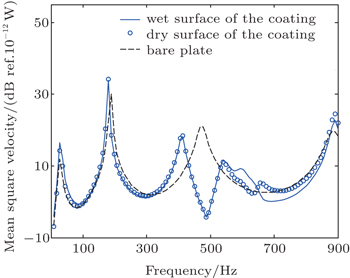Fig. 4. Mean square velocity of the dry/wet surface.

The base layer’s velocity of order m without decoupling layer can be expressed as: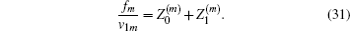From Eq. (25), the model velocity of the base layer with decoupling layer can also be expressed as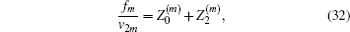where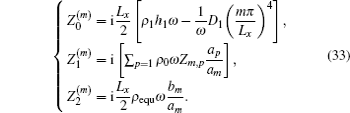In Eqs. (31) and (32),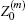is the base plate’s mechanical impedance which is only decided by base plate itself.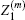and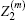are system’s additional mechanical impedance which is decided by water and decoupling layer. Figure 5(a) shows the change of the impedance by calculating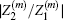. The calculation result indicates that decoupling layer greatly increases system’s additional mechanical impedance which causes the suppressing of system’s vibrations around 490 Hz compared to bare plate.

In Eqs. (25) and (33), we can observe that the change of additional mechanical impedance of system is directly related to effective density ρequ. Figure 5(b) shows the change of effective density of the decoupling layer. The result indicates that ρequ has an obvious break around 490 Hz corresponding to the change of system’s velocity, and ρequ also shows a distinct characteristic of metamaterial around 490 Hz with real part’s change from 1.58×104 kg/cm3 to −1.09×104 kg/cm3 and large imaginary part’s peak near −2.67×104 kg/cm3. This characteristic is always related to the resonance of metamaterial which often leads to transformation in acoustic properties.[21,22]

 Figure Option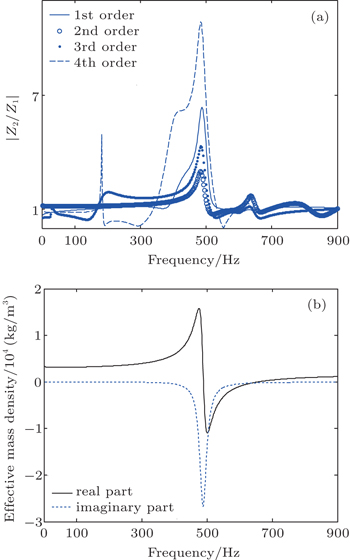Fig. 5. The change of (a) the mechanical impedance and (b) effective mass density of the decoupling layer.
3.3. The characteristic of resonant model

Figures 6(a) and 6(b) show the model of plane wave transmission and the model of sound radiation of systems at 490 Hz. The result indicates that locally resonant structure in two models possesses similar resonant modality in which the core and coating of the resonant constitute vibration mode as spring oscillator and the base material almost keeps still compared to the coating. The parts of the coating which are beside the core has an effect like shear springs. The top and bottom parts have an effect like compressing-extension springs. This resonant mode could greatly restrain base plate’s vibration like dynamic vibration absorber’s effect at resonant frequency. While system’s noise reduction is the special properties of decoupling layer and has little relation with the base plate, so the noise reduction of decoupling layer should be suitable to different base plates. Figure 7 shows mean square velocity of plates in different sizes which are covered with decoupling layer mentioned above. The result indicates that all the covered plates’ velocities have an obvious valley around 490 Hz.

Now that system’s vibration can be restrained by decoupling layer with periodic LRS, the noise reduction’s frequency could also be regulated on the basis of the LRS’s characteristic. Figure 6(c) show the model which is used for quickly calculating the resonant frequency of LRS. This model is a cell of the decoupling layer with the top and bottom’ z-direction velocity limited to zero. So this simple model could reflect LRS’s resonant mode in z direction by calculating the model’s nature frequency.

 Figure Option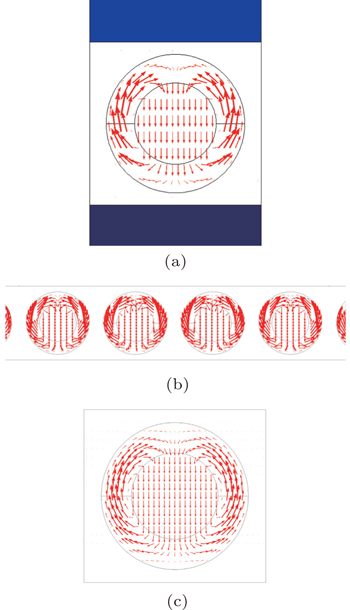Fig. 6. The displacement amplitude fields at resonant frequency (a) transmission coefficient model, (b) radiation model, (c) natural frequencies model.
 Figure Option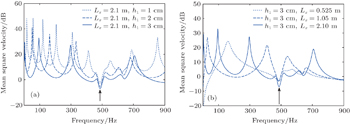Fig. 7. Mean square velocity of dry surface of the system with (a) different thicknesses and (b) different lengths.

Figure 8 shows the resonant frequency of the three models in Fig. 6 with different material properties of the LRS. The results indicate the frequencies calculated by three methods coincide well with each other. But the estimate for resonant frequency by simple model is more convenient than the others since it just needs calculating the modality’s corresponding frequencies directly, while the others need calculating the transmission coefficient or radiation power of the models with and without LRS in a wide range of the frequencies. And the results indicate that the resonant frequency decreases as the density of the core increases and it increases as the transverse wave velocity of the coating increases.

 Figure Option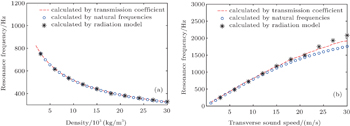Fig. 8. Resonance frequency with different (a) density and (b) transverse wave velocity.
4. Conclusions

This paper presents an analysis of vibration and sound radiation influence of the decoupling layer with periodic LRS. A theoretical calculation model for the decoupled plate with periodic LRS has been developed based on the use of effective medium theory and elastodynamics theory. Such a model is more efficient than conventional finite element model and shows adequate accuracy for acoustic analysis in preliminary design. The numerical analysis gives the following findings:

Decoupling layer with LRSs can significantly reduce the sound radiation of underwater plates around the LRS’s resonance frequency. The performance of sound radiation reduction is mostly caused by the suppression of base plate vibration.

The additional mechanical impedance of the system is directly dependent on the effective density of decoupling layer. The shake-up of effective density around local resonance frequency greatly effects system’s mechanical impedance and thus suppresses the vibration of base plate. The vibration suppression properties of decoupling layer could be guaranteed for different sizes of base plate.

The locally resonant structures involved in the radiation prediction model and transmission prediction model, have similar vibration modalities. A simple model can be adopted to predict the local resonance frequency of the decoupling layer with LRS. The local resonance frequency decreases as the density of the core increases and increases as the transverse wave velocity of the coating increases.

Reference
 1 Ko S H 1997 J. Acoust. Soc. Am. 101 3306 2 Berry AFoin O 2001 J. Acoust. Soc. Am. 109 2704 3 Wang X ZZhang A MPang F ZYao X L 2014 J. Sound Vib. 333 228 4 Tao MTang W LHua H X 2010 J. Vib. Acoust. 132 61001 5 Zhu D WHuang X CWang YXiao FHua H X2014J. Mechan-ical Engineering Science41622014 6 Huang L ZXiao YWen J HYang H BWen X S 2015 Acta Phys. Sin. 64 154301 (in Chinese) 7 Liu Z YZhang X XMao Y WZhu Y YYang Z YChan C TSheng P 2000 Science 289 1734 8 Mei JLiu Z YShi JTian D C 2003 Phys. Rev. B 67 245107 9 Liu Z YChan C TSheng P 2000 Phys. Rev. B 62 2446 10 Meng HWen J HZhao H GLv L MWen X S 2012 J. Acoust. Soc. Am. 132 69 11 Meng HWen J HZhao H GWen X S 2012 J. Sound Vib. 2012 4406 12 Zhao H GWen J HYu D LWen X S 2010 J. Appl. Phys. 107 23519 13 Wen J HZhao H GLv L MYuan BWang GWen X S 2011 J. Acoust. Soc. Am. 130 1201 14 Jiang HWang YZhang MHu YLan DZhang YWei B 2009 Appl. Phys. Lett. 95 104101 15 Duan Y TLuo JWang G HHang Z HHou BLi J SSheng PLai Y 2015 Sci. Rep. 5 12139 16 Zhao H GWen J HYang H BLv L MWen X S 2014 Acta Phys. Sin. 63 134303 (in Chinese) 17 Hladky-Hennion A CDecarpigny J N 1991 J. Acoust. Soc. Am. 90 3356 18 Foin OBerry A 2000 J. Acoust. Soc. Am. 107 2501 19 Still TOudich MAuerhammer G KVlassopoulos DDjafari-Rouhani BFytas GSheng P 2013 Phys. Rev. B 88 94102 20 He S PHe Y AZhang W Q2014Acta Acoust. 39177(in Chinese) 21 Fokin VAmbati MSun CZhang X 2007 Phys. Rev. B 76 144305 22 Zhou X MHu G K 2009 Phys. Rev. B 79 195109